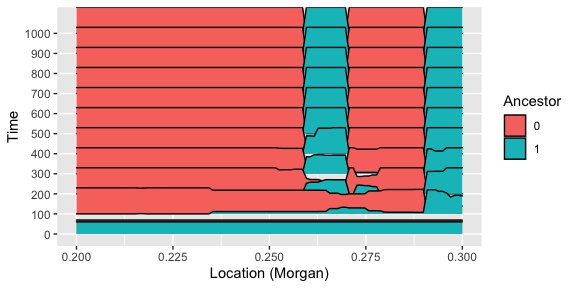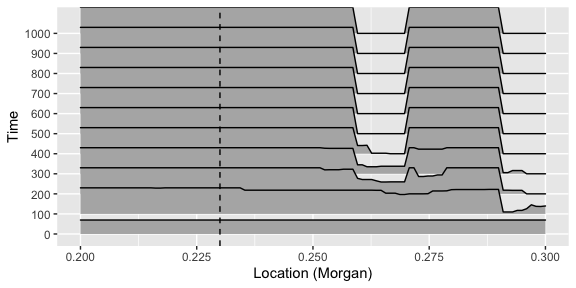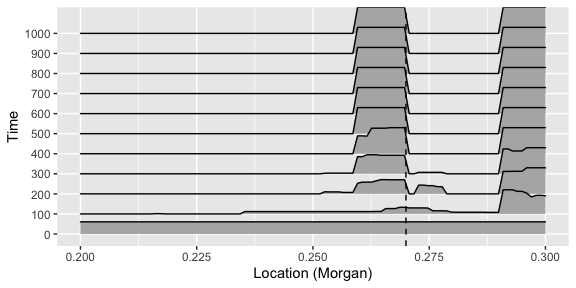# Demonstrate joyplot

library(GenomeAdmixR)
library(ggplot2)
packageVersion("GenomeAdmixR")
##  '2.1.7'

# Demonstration of how to use joyplots

To demonstrate the applicability and versatility of joyplots, we create a population, and select on this population on two separate locations, for two different ancestors.

select_matrix <- matrix(ncol = 5, nrow = 2)
s <- 0.1
select_matrix[1, ] <- c(0.23, 1.0, 1 + 0.5 * s, 1 + s, 0)
select_matrix[2, ] <- c(0.27, 1.0, 1 + 0.5 * s, 1 + s, 1)

markers <- seq(from = 0.2, to = 0.3, length.out = 100)

We track frequencies of all ancestors in the range [0.2, 0.3], using 100 markers.

selected_pop <- simulate_admixture(
module = ancestry_module(number_of_founders = 2,
morgan = 1,
markers = markers),
pop_size = 100,
total_runtime = 1001,
select_matrix = select_matrix)
## Found a selection matrix, performing simulation including selection

We can now plot all the ancestors over time, we choose here to plot them only for a select subset of times.

time_points <- seq(from = 0, to = 1000, by = 100)
plot_joyplot_frequencies(selected_pop$frequencies, time_points, picked_ancestor = "ALL")Alternatively, we can plot them separately. p1 <- plot_joyplot_frequencies(selected_pop$frequencies,
time_points,
picked_ancestor = 0)
p1 + ggplot2::geom_vline(xintercept = 0.23, lty = 2)And for the other ancestor:

p2 <- plot_joyplot_frequencies(selected_pop\$frequencies,
time_points,
picked_ancestor = 1)
p2 + ggplot2::geom_vline(xintercept = 0.27, lty = 2)